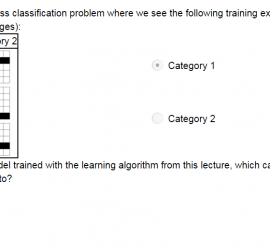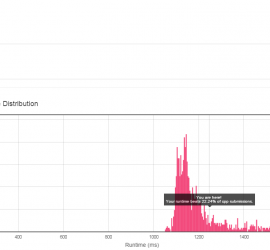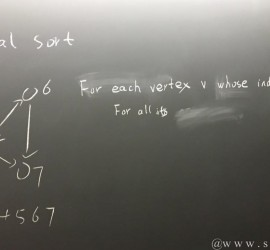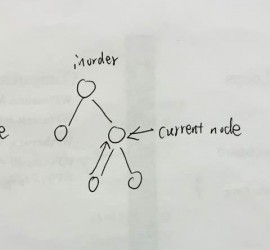# Yearly Archives: 2015

## [Coursera] Neural Networks for Machine Learning

This is the note for the course Neural Networks in Coursera. Course Link: https://class.coursera.org/neuralnets-2012-001/lecture Why do we need machine learning In a typical program, we define a lot of rules, which are represented by ‘loop’, ‘if condition’ and ‘assignment’. If the program is correct, given some inputs, we can always get […]## [leetcode] Coin Change2

322. Coin Change You are given coins of different denominations and a total amount of money amount. Write a function to compute the fewest number of coins that you need to make up that amount. If that amount of money cannot be made up by any combination of the coins, […]

## [leetcode] 310. Minimum Height Trees1

Minimum Height Trees For a undirected graph with tree characteristics, we can choose any node as the root. The result graph is then a rooted tree. Among all possible rooted trees, those with minimum height are called minimum height trees (MHTs). Given such a graph, write a function to find […]## Vermont滑雪## 刷题总结3## How to convert recursion algorithms into iteration version1

In technical interviews, many candidates are asked to implement recursion algorithms using iteration. Generally, an algorithm implemented in iteration will run faster than that in recursion, since it avoids the overhead of function call. With the help of a stack and careful algorithm design, we can convert all recursion algorithms […]